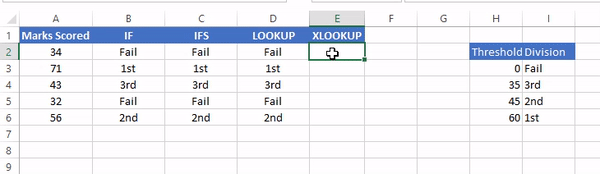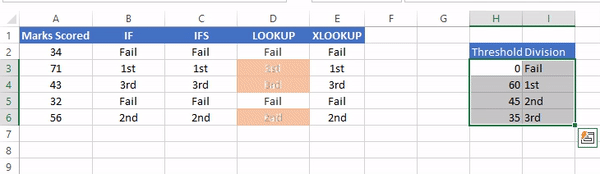top of page
SearchHow many of you use Nested IFs in Excel? Mastering Nested IFs takes time and effort, especially those who are not savvy with formulas. Somehow you managed to write Nested IFs, but when it comes to editing or making modifications, then it looks like a challenge—struggling with proper brackets and conditions. Complicated at times :)

Well, there is good news. Modern Excel (Office 365 & Excel 2016 -19) has tamed this beast in multiple ways. In this article, we are going to see a couple of them.

I am hoping that you are familiar with IF.

This function follows a typical IF (Condition is TRUE) THEN (Result/Action 1) ELSE (Result/Action 2) logic. In the case of multiple conditions, we must use the IF function multiple times. Let us take a simple example:

Following table stands for the divisions based on the marks scored in the exam:## Nested IFs using AND (The traditional approach)

In traditional Excel, the following would have been the possible solution:

`=IF(A2<35,"Fail",IF(AND(A2>=35,A2<45),"3rd",IF(AND(A2>=45,A2<60),"2nd","1st")))`Question: How many "IF" needed in the traditional nested ifs?

The thumb rule is: If the number of categories is n, then if need to supply (n-1) IF. In this example, there are four categories (Fail, 3rd, 2nd, and 1st). Hence, we need (4-1) = 3 IF. The last category comes under else output of the last IF statement.

This approach is a little complicated, especially those who are relatively new at Excel.

## Nested IF using IFS (The modern approach)

In Modern Excel, we have something called IFS and here how it has replaced the traditional IF:

`=IFS(A8<35,"Fail",A8<45,"3rd Division",A8<60,"2nd Division",A8>=60,"1st Division")`IFS function is 23% shorter than the traditional Nested IF (in terms of length of the formula) and relatively easy to write. No need to worry about combining IF, AND, and OR.

One thing which IFS required is that you cover all the ground of logic. In this example, we need to tell IFS function what value to return for Marks Scored >= 60 as well. It was not the case in the traditional IF statement.

## Nested IF using LOOKUP (The smart approach)

There is another smart way to handle such a scenario: using LOOKUP. Not VLOOKUP or HLOOKUP, but just LOOKUP.

All you need to do is create a simple table with the threshold defined for each level (as shown in the table below) and then apply the formula at the desired cell:

`=LOOKUP(A2,\$G\$3:\$H\$6)`Voila! That's it.

LOOKUP has two segments

LOOKUP_VALUE: The cell having the value. In this example, A8 is the cell having the mark

ARRAY: The range of the table. In this example, G3:H6. Do not include the header.

You need to take care of the following points:

· The threshold should be the start point of the level. So, in this example, the Fail starts from 0, 3rd division starts from 35, so on and so forth.

· Fix the table range by using \$ signs as there is a high probability that you would be copy-pasting this formula in multiple cells. In this example, I have fixed the array A2:B5 to \$A\$2:\$B\$5

## Nested IF using XLOOKUP (The smarter approach)

One of the latest entrants in formula and arguably very versatile. It has gained significant popularity among Excel users due to a wide range of options it offers.

XLOOKUP has the following syntax:

Lookup_value: What you are looking for

Lookup_array: Where you are looking for

Return_array: The output column you need if the match found

If_not_found (optional): What to return if no matches found

Match_mode (optional): By default, it selects "0-Exact Match", but it offers the following added options:So, our formula is:

`=XLOOKUP(A2,\$H\$3:\$H\$6,\$I\$3:\$I\$6,,-1)`Applying XLOOKUP for distributing data into categories

XLOOKUP advantage over LOOKUP: LOOKUP requires the reference table to sorted in ascending order for correct output, while XLOOKUP doesn't.So, which one do you prefer?

Related article: Adding Categories with Power Query

@imVivRan

bottom of page